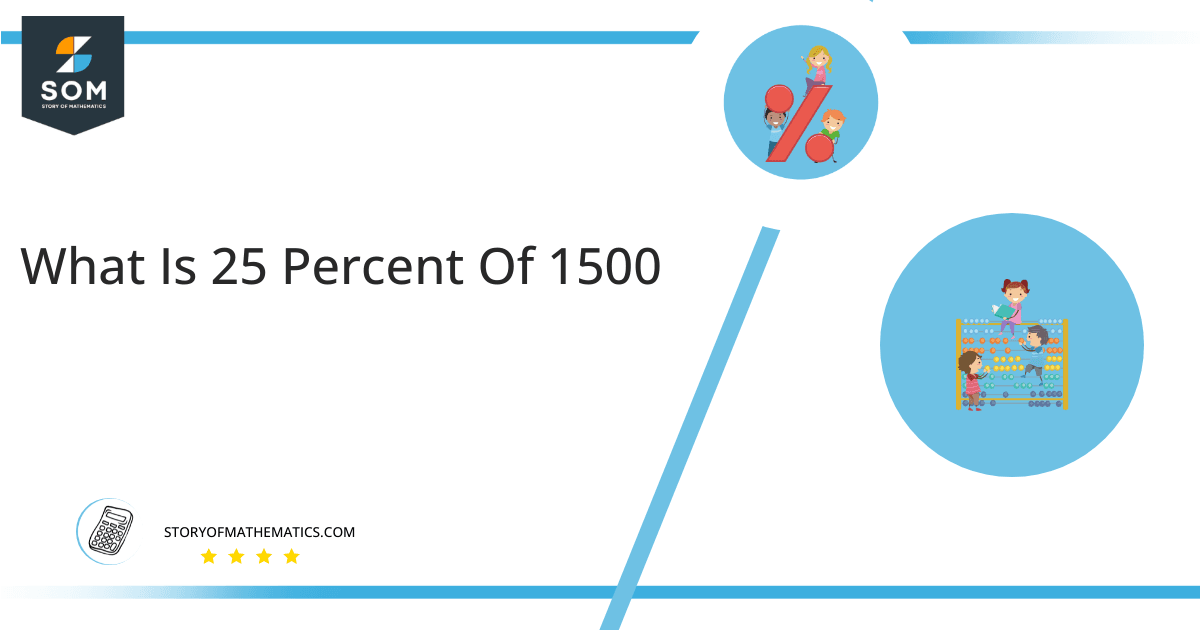# What Is 25 Percent of 1500 + Solution with Free Steps?The 25 percent of 1500 is equal to 375. It can be easily calculated by dividing 25 by 100 and multiplying the answer with 1500 to get 375.

The easiest way to get this answer is by solving a simple mathematical problem of percentage. You need to find 25% of 1500for some sale or real-life problem. Divide 25 by 100, multiply the answer with 1500, and get the 25% of 1500 value in seconds.

This article will explain the full process of finding any percentage value from any given quantity or number with easy and simple steps.

## What Is 25 percent of 1500?

The 25 percent of 1500 is 375.

The percentage can be understood with a simple explanation. Take 1500, and divide it into 100 equal parts. The 25 number of parts from the total 100 parts is called 25 percent, which is 375 in this example.

## How To Calculate 25 percent of 1500?

You can find 25 percent of 1500 by some simple mathematical steps explained below.### Step 1

Firstly, depict 25 percent of 1500 as a fractional multiple as shown below:

25% x 1500

### Step 2

The percentage sign % means percent, equivalent to the fraction of 1/100.

Substituting this value in the above formula:

= (25/100) x 1500

### Step 3

Using the algebraic simplification process, we can arithmetically manipulate the above equation as follows:

= (25 x 1500) / 100

= 37500 / 100

= 375This percentage can be represented on a pie chart for visualization. Let us suppose that the whole pie chart represents the 1500 value. Now, we find 25 percent of 1500, which is 375. The area occupied by the 375 value will represent the 25 percent of the total 1500 value. The remaining region of the pie chart will represent 75 percent of the total 1500 value. The 100% of 1500 will cover the whole pie chart as 1500 is the total value.

Any given number or quantity can be represented in percentages to better understand the total quantity. The percentage can be considered a quantity that divides any number into hundred equal parts for better representation of large numbers and understanding.

Percentage scaling or normalization is a very simple and convenient method of representing numbers in relative terms. Such notations find wide application in many industrial sectors where the relative proportions are used.